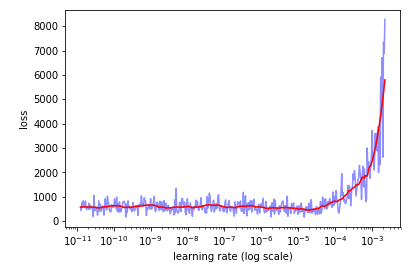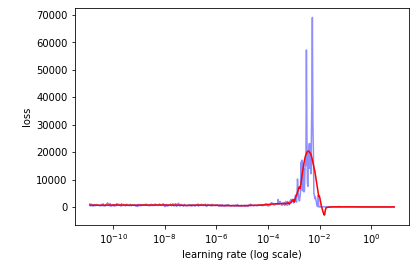# Learning Rate Finder: Loss doesn't decrease?

I’m trying to implement the learning rate finder which is what Jeremy Howard uses in Fast.ai on a 3D convolutional neural network in Keras. Here are two resources for reference:

In brief, I am trying to predict a 3D bounding box, so this is a regression problem with 6 outputs (x1,y1,z1,x2,y2,z2). The idea is to slowly increment the learning rate until the loss explodes. The inflection point sets the maximum range for the learning rate that you want to use. However, my results don’t seem to follow this idea. The loss never seems to dip, unless I make the stopping condition larger.

The second link is the code that I am trying to use for the LR finder. My network is as follows:

``````# Basic Feature Extractor
x = Conv3D(filters=32, kernel_size = (3, 3, 3), strides=(1,1,1), padding='same', \
activation='relu', name='block1_conv1',kernel_initializer='random_normal')
(img_input)
x = Conv3D(filters=32, kernel_size = (3, 3, 3), strides=(1,1,1), padding='same', \
activation='relu', name='block1_conv2',kernel_initializer='random_normal')(x)
x = Conv3D(filters=64, kernel_size = (3, 3, 3), strides=(1,1,1), padding='same', \
activation='relu', name='block1_conv3',kernel_initializer='random_normal')(x)
x = Conv3D(filters=64, kernel_size = (3, 3, 3), strides=(1,1,1), padding='same', \
activation='relu', name='block1_conv4',kernel_initializer='random_normal')(x)
x = Conv3D(filters=128, kernel_size = (3, 3, 3), strides=(1,1,1), padding='same', \
activation='relu', name='block1_conv5',kernel_initializer='random_normal')(x)
x = BatchNormalization(name='bn_5')(x)
x = Conv3D(filters=128, kernel_size = (3, 3, 3), strides=(1,1,1), padding='same', \
activation='relu', name='block1_conv6',kernel_initializer='random_normal')(x)
x = Flatten(name='flatten')(x)
out = Dense(6, activation='linear', kernel_initializer='random_normal', name='regr_output')(x)
``````

For reference, this model does pretty well on my dataset (aside from the overfitting). Here’s a loss vs epoch curve on train and validation :

I’m trying to find a more optimal learning rate. In the results above I used a constant learning rate of 1e-5 which I picked arbitrarily to start off with.

Starting at a learning rate of 1e-11 and incrementing it over a batch size of 64, 5 epochs, and dataset size of 10,000 images, my loss vs learning rate plot never decreases:It isn’t until I bump up my stopping condition that the loss goes down, but at this point i’m assuming that my model has already learned enough to decrease the loss.Does this mean that my model is not as sensitive to the learning rate, or is something going wrong here?

Did you do this on a pre-trained model or everything is init from scratch?
I face the same situation when run lr_finder on a pre-trained model before fine-tune. However if I run it on a newly init model, it’s works OK.